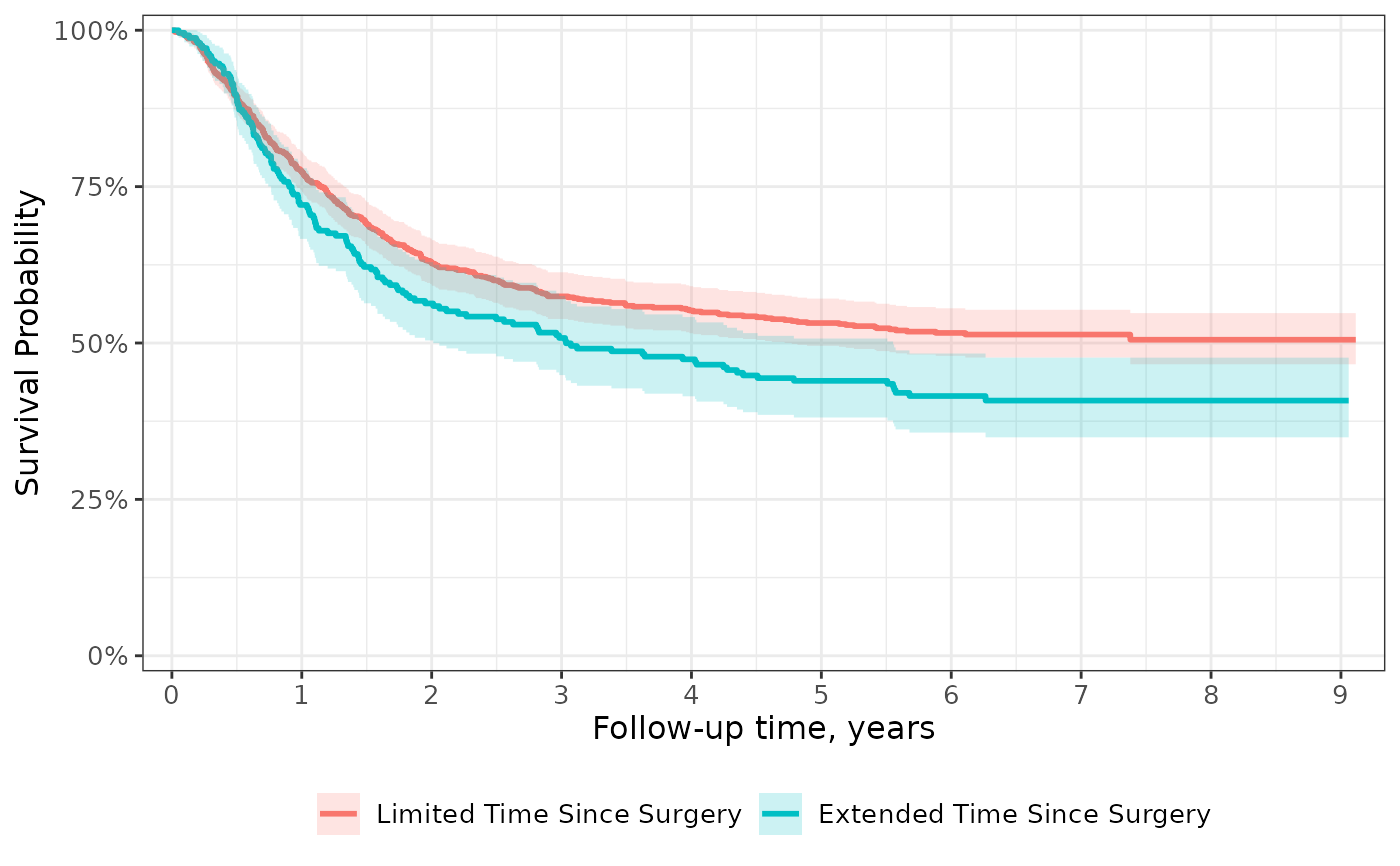The most common figure created with this package is a survival curve. This scale applies modifications often seen in these figures.

• scale_y_continuous(expand = c(0.025, 0), limits = c(0, 1), label = scales::label_percent()).

• scale_x_continuous(expand = c(0.015, 0), n.breaks = 8)

If you use this function, you must include all scale specifications that would appear in scale_x_continuous() or scale_y_continuous(). For example, it's common you'll need to specify the x-axis break points. scale_ggsurvfit(x_scales=list(breaks=0:9)).

To reset any of the above settings to their ggplot2 default, set the value to NULL, e.g. y_scales = list(limits = NULL).

## Usage

scale_ggsurvfit(x_scales = list(), y_scales = list())

## Arguments

x_scales

a named list of arguments that will be passed to ggplot2::scale_x_continuous().

y_scales

a named list of arguments that will be passed to ggplot2::scale_y_continuous().

## Value

a ggplot2 figure

Visit the gallery for examples modifying the default figures

## Examples

ggsurvfit <-
survfit2(Surv(time, status) ~ surg, data = df_colon) %>%
ggsurvfit(linewidth = 1) +